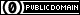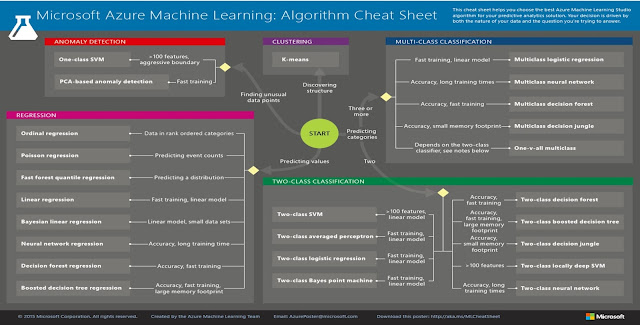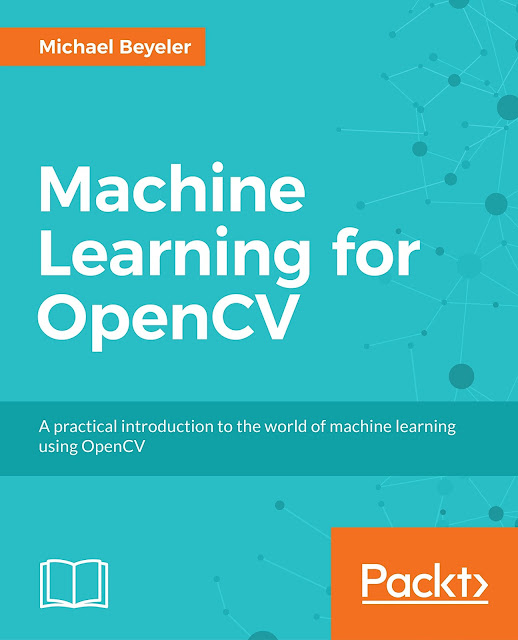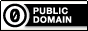## Saturday, February 25, 2017### How to choose the right algorithm for your machine learning problem

With the recent machine learning boom, more and more algorithms have become available that perform exceptionally well on a number of tasks. But knowing beforehand which algorithm will perform best on your specific problem is often not possible. If you had infinite time at your disposal, you could just go through all of them and try them out. The following post shows you a better way to do this, step by step, by relying on known techniques from model selection and hyper-parameter tuning.### Step 0: Know the basics

Before we get in too deep, we want to make sure we brushed up on the basics. In specific, we should know that there are three main categories of machine learning: supervised learning, unsupervised learning, and reinforcement learning.

•In supervised learning, each data point is labeled or associated with a category or value of interest. An example of a categorical label is assigning an image as either a "cat" or a "dog". An example of a value label is the sale price associated with a used car. The goal of supervised learning is to study many labeled examples like these, and then to be able to make predictions about future data points—for example, to identify new photos with the correct animal (classification) or to assign accurate sale prices to other used cars (regression).
• In unsupervised learning, data points have no labels associated with them. Instead, the goal of an unsupervised learning algorithm is to organize the data in some way or to describe its structure. This can mean grouping it into clusters, or finding different ways of looking at complex data so that it appears simpler.
• In reinforcement learning, the algorithm gets to choose an action in response to each data point. It is a common approach in robotics, where the set of sensor readings at one point in time is a data point, and the algorithm must choose the robot's next action. It's also a natural fit for Internet of Things applications, where the learning algorithm receives a reward signal a short time later, indicating how good the decision was. Based on this, the algorithm modifies its strategy in order to achieve the highest reward.### Step 1: Categorize the problem

Next up, we want to categorize the problem at hand. This is a two-step process:

• Categorize by input: If we have labeled data, it's a supervised learning problem. If we have unlabeled data and want to find structure, it's an unsupervised learning problem. If we want to optimize an objective function by interacting with an environment, it's a reinforcement learning problem.
• Categorize by output: If the output of our model is a number, it's a regression problem. If the output of our model is a class (or category), it's a classification problem. If the output of our model is a set of input groups, it's a clustering problem.

It's as simple as that.

More generally speaking, we can find the right category of algorithms by asking ourselves what our algorithm is trying to achieve:

The above illustration contains a few technical terms we haven't talked about yet:

• Classification: When the data are being used to predict a category, supervised learning is also called classification. This is the case when assigning an image as a picture of either a "cat" or a "dog". When there are only two choices, this is called two-class or binomial classification. When there are more categories, as when predicting the winner of the next Nobel Prize in Physics, this problem is known as multi-class classification.
• Regression: When a value is being predicted, as with stock prices, supervised learning is called regression.
• Clustering: One of the most common approaches to unsupervised learning is called cluster analysis or clustering. Clustering is the task of grouping a set of objects in such a way that objects in the same group (called a cluster) are more similar (in some sense or another) to each other than to those in other groups (clusters).
• Anomaly detection: Sometimes the goal is to identify data points that are simply unusual. In fraud detection, for example, any highly unusual credit card spending patterns are suspect. The possible variations are so numerous and the training examples so few, that it's not feasible to learn what fraudulent activity looks like. The approach that anomaly detection takes is to simply learn what normal activity looks like (using a history of non-fraudulent transactions) and identify anything that is significantly different.

### Step 2: Find the available algorithms

Now that we have categorized the problem, we can identify the algorithms that are applicable and practical to implement using the tools at our disposal.

Microsoft Azure has created a handy algorithm cheat sheet that shows which algorithms can be used for which category of problems. Although the cheat sheet is tailored towards Azure software, it is generally applicable:Click the above image to enlarge, or download the original PDF.

A few notable algorithms are:

• Classification:
• Support vector machines (SVMs) find the boundary that separates classes by as wide a margin as possible. When the two classes can't be clearly separated, the algorithms find the best boundary they can. Where it really shines is with feature-intense data, like text or genomic (> 100 features). In these cases, SVMs are able to separate classes more quickly and with less overfitting than most other algorithms, in addition to requiring only a modest amount of memory.
• Artificial neural networks are brain-inspired learning algorithms covering multiclass, two-class, and regression problems. They come in an infinite variety, including perceptrons and deep learning. They take a long time to train, but are known to deliver state-of-the-art performance in a variety of application fields.
• Logistic regression: Although it confusingly includes 'regression' in the name, logistic regression is actually a powerful tool for two-class and multiclass classification. It's fast and simple. The fact that it uses an 'S'-shaped curve instead of a straight line makes it a natural fit for dividing data into groups. Logistic regression gives linear class boundaries, so when you use it, make sure a linear approximation is something you can live with.
• Decision trees and random forests: Decision forests (regression, two-class, and multiclass), decision jungles (two-class and multiclass), and boosted decision trees (regression and two-class) are all based on decision trees, a fundamental machine learning concept. There are many variants of decision trees, but they all do the same thing—subdivide the feature space into regions with mostly the same label. These can be regions of consistent category or of constant value, depending on whether you are doing classification or regression.

• Regression:
• Linear regression fits a line (or plane, or hyperplane) to the data set. It's a workhorse, simple and fast, but it may be overly simplistic for some problems.
• Bayesian linear regression has a highly desirable quality: it avoids overfitting. Bayesian methods do this by making some assumptions beforehand about the likely distribution of the answer. Another byproduct of this approach is that they have very few parameters.
• Boosted decision tree regression: As mentioned above, boosted decision trees (regression and two-class) are based on decision trees, and work by subdividing the feature space into regions with mostly the same label. Boosted decision trees avoid overfitting by limiting how many times they can subdivide and how few data points are allowed in each region. The algorithm constructs a sequence of trees, each of which learns to compensate for the error left by the tree before. The result is a very accurate learner that tends to use a lot of memory.
• Clustering:
• Hierarchical clustering seeks to build a hierarchy of clusters, and it comes in two flavors. Agglomerative clustering is a "bottom up" approach, where each observation starts in its own cluster, and pairs of clusters are merged as one moves up the hierarchy. Divisive clustering is a "top down" approach, where all observations start in one cluster, and splits are performed recursively as one moves down the hierarchy. In general, the merges and splits are determined in a greedy manner. The results of hierarchical clustering are usually presented in a dendrogram.
• k-means clustering aims to partition n observations into k clusters in which each observation belongs to the cluster with the nearest mean, serving as a prototype of the cluster. This results in a partitioning of the data space into Voronoi cells.
• Anomaly detection:
• k-nearest neighbors (or k-NN for short) is a non-parametric method used for classification and regression. In both cases, the input consists of the k closest training examples in the feature space. In k-NN classification, the output is a class membership. An object is classified by a majority vote of its neighbors, with the object being assigned to the class most common among its k nearest neighbors (k is a positive integer, typically small). In k-NN regression, the output is the property value for the object. This value is the average of the values of its k nearest neighbors.
• One-class SVM: Using a clever extension of nonlinear SVMs, the one-class SVM draws a boundary that tightly outlines the entire data set. Any new data points that fall far outside that boundary are unusual enough to be noteworthy.

### Step 3: Implement all of the applicable algorithms

For any given problem, there are usually a handful of candidate algorithms that could do the job. So how do we know which one to pick? Often, the answer to this problem is not straightforward, so we have to resort to trial-and-error.

Prototyping is best done in two steps. In the first step, we want to have some quick-and-dirty implementation of several algorithms with minimal feature engineering. At this stage, we are mainly interested in seeing which algorithm behaves better at a coarse scale. This step is a bit like hiring: We're looking for any reason to shorten our list of candidate algorithms.

Once we reduced the list to a few candidate algorithms, the real prototyping begins. Ideally, we would want to set up a machine learning pipeline that compares the performance of each algorithm on the dataset using a set of carefully selected evaluation criteria. At this stage, we are only dealing with a handful of algorithms, so we can turn our attention to where the real magic lies: feature engineering.

### Step 4: Feature engineering

Perhaps even more important than choosing the right algorithm is choosing the right features to represent the data. Whereas it can be relatively straightforward to select a suitable algorithm from the list presented above, Feature engineering is more of an art.

The main problem is that the data we are trying to classify is rarely described in the most informative feature space: for example, grayscale values of pixels are a poor predictor of what an image depicts. Instead we need to find data transformations that improve the signal and reduce noise. Without these transformations, the task at hand might be unsolvable. For example, before the advent of histogram of oriented gradients (HOG), intricate visual tasks such as pedestrian detection or face detection were really hard to do.

Although the validity of most features might only be assessed by trial-and-error, it is good to know about common ways to discover informative features in your data. Among the most prominent techniques are:

• Principal component analysis (PCA): A linear dimensionality reduction technique that can be used to find a small number of highly informative principal components, which can explain most of the variance in the data.
• Scale-invariant feature transform (SIFT): A patented algorithm in computer vision to detect and describe local features in images. It has an open-source alternative called ORB.

• Speeded up robust features (SURF): A patented and more robust version of SIFT.
• Histogram of oriented gradients (HOG): A feature descriptor used in computer vision to count occurrences of gradient orientation in localized portions of an image.
• ...and many more described here.

Of course, you can also come up with your own feature descriptor. If you have a couple of candidates, you can perform smart feature selection using a wrapper method:

• Forward search:
• Greedily include the most relevant feature: Add a candidate feature to your existing feature set and calculate the model's cross-validation error. Repeat for all other candidate features. In the end, add the candidate feature that yielded the lowest error.
• Repeat until the desired number of features is selected.
• Backward search:
• Greedily remove the least relevant feature. Remove a candidate feature from your existing feature set and calculate the model's cross-validation error. Repeat for all other candidate features. In the end, remove the candidate feature that yielded the largest error improvement.
• Repeat until the desired number of features is selected.

Always use cross-validation for inclusion/removal criteria!

### Step 5: Optimize hyperparameters (optional)

Finally, you also want to optimize an algorithm's hyperparameters. Examples might include the number of principal components of PCA, the parameter k in the k-nearest neighbor algorithm, or the number of layers and learning rate in a neural network. This is again best done using cross-validation.

Once you put all of these steps together, you have good chances of having created a very powerful machine learning system. But, as you might have already guessed: the devil is in the details, and you might have to resort to trial-and-error.

Good luck!More information can be found in the book Machine Learning for OpenCV, Packt Publishing Ltd., July 2017.To the extent possible under law, Michael Beyeler has waived all copyright and related or neighboring rights to "How to choose the right algorithm for your machine learning problem".